# Graphs using MySQL data & DataFrame

Pandas plotThere are three steps in reading data and displaying graph.
1. Connect to MySQL Database
2. Get Data using Query and Create DataFrame
3. Plotting of Graph

## Connect to MySQL Database

Using SQLAlchemy we will connect to MySQL database, replace your userid , password and database name ( db_name ) .
``````from sqlalchemy import create_engine
We will use the connection string object my_conn in our query.

## Get Data using Query and Create DataFrame

SQL query is the first step to get data from MySQL database. This query can be changed to get different type / form of data. We used read_sql() to create the DataFrame using returned data from MySQL table.
``````query="SELECT class,COUNT( * ) number FROM student GROUP BY class"
lb= [row for row in df['class']] # Labels of graph``````

## Plotting of Graph

``````plot=df.plot.pie(title="Students",y='number',
labels=lb,autopct='%1.0f%%')``````
Full code is here.
``````import pandas as pd # import Pandas library
from sqlalchemy import create_engine
query="SELECT class,COUNT( * ) number FROM student GROUP BY class"
lb= [row for row in df['class']] # Labels of graph

plot=df.plot.pie(title="Students ",y='number',labels=lb,autopct='%1.0f%%')``````

## Using Bar graph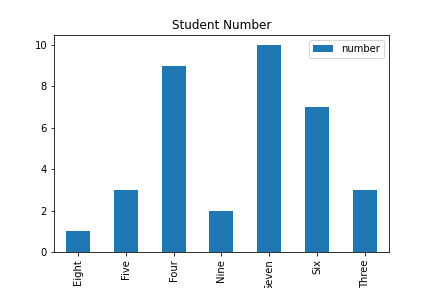Connection to MySQL and query execution remain same as above code, only the last line is changed to generate the bar graph.
``````import pandas as pd
from sqlalchemy import create_engine
my_conn = create_engine("mysql+mysqldb://root:test@localhost/my_tutorial")
query="SELECT class,COUNT(*) number FROM student GROUP BY class"
plot=df.plot.bar(title="Student Number",x='class');``````

## Horizontal Bar graph

We will plot one Horizontal bar graph by changing the last line only.
``plot=df.plot.barh(title="Student Number",x='class');``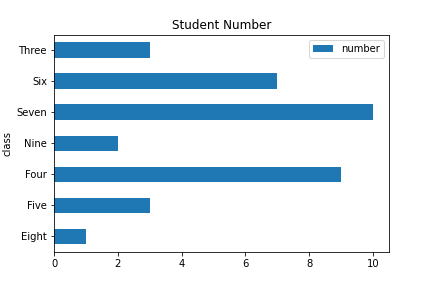## More than one value in Bar graph

In above code we kept two columns, now we will use three columns. One columns class we will use in y axis, other two columns maximum mark ( max ) and average value ( avg ) will be used to draw the graphs.

Note that only the Query part is changed ( from above code ) here and the DataFrame is having one more column data
``````import pandas as pd # import Pandas library
from sqlalchemy import create_engine
my_conn = create_engine("mysql+mysqldb://root:test@localhost/my_tutorial")
query="SELECT class,MAX(mark) max, AVG(mark) avg FROM student GROUP BY class"
plot=df.plot.bar(title="Student Number",x='class');``````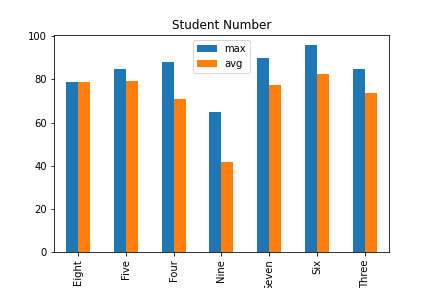## Stacked Bar Graph

We will break the number of students in two parts based on gender ( male , female ) and create stacked bar graph ( Note the option stacked=True ). Query is also changed to include GROUP BY and CASE.
``````import pandas as pd # import Pandas library
from sqlalchemy import create_engine
my_conn = create_engine("mysql+mysqldb://root:test@localhost/my_tutorial")
query="SELECT class,\
sum(CASE WHEN gender ='male' THEN 1 ELSE 0 END) as Male,\
sum(CASE WHEN gender ='Female' THEN 1 ELSE 0 END) as Female\
FROM student group by class"
plot=df.plot.bar(title="Student Number",x='class',stacked=True);``````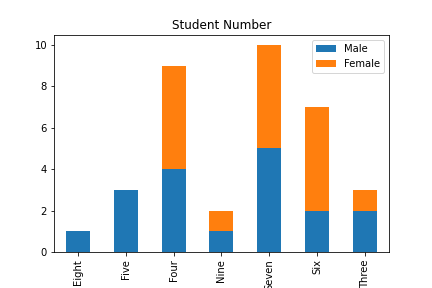## Using Line Graph

Only the last line is changed ( from the above code given for simple bar graph) .
``plot=df.plot.line(title="Student Number",x='class',y='number')``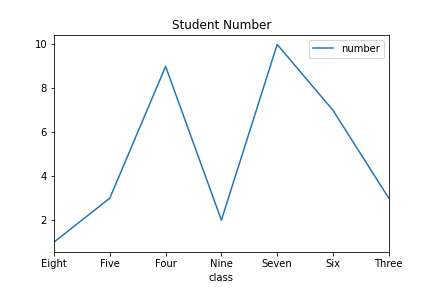## Density graph

Using mark column we will create density graph. The query and drawing graph is only shown here as the library and connection string is same as given above.
``````query="SELECT mark FROM student"
plot=df.plot.density();``````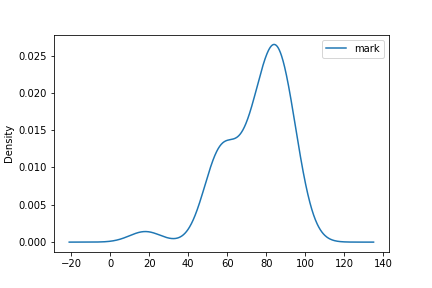## Scatter graph

Two numeric columns are used for this graph.
``````query="SELECT COUNT( * ) number,avg(mark) avg FROM student GROUP BY class"
plot=df.plot.scatter(title="Average Vs Number ",x='avg',y='number')``````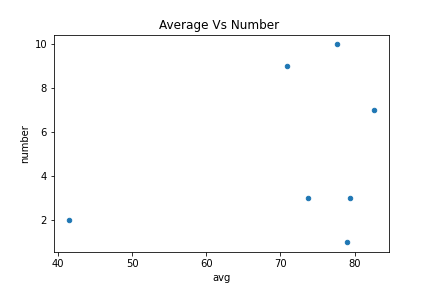## Saving graph as image

``````plot=df.plot.pie(title="Students",y='number',
labels=lb,autopct='%1.0f%%')
fig = plot.get_figure()
fig.savefig("D:\\my_data\\output2.png")``````

Subscribe to our YouTube Channel here

## Subscribe

* indicates required
Subscribe to plus2netplus2net.com